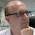Monday, 1 November 2010

Functional Programming Challenge: Most Frequent Multiple Occurring List Item

A little functional programming challenge for those who like that sort of thing. This one came up while I was improving my Noughts and Crosses example application.

The challenge is this: Given a list of elements of type T, find the element with the most multiple occurrences.

For example, the following should apply:

```List("a", "b", "c", "a", "b", "a")
-> should return "a" as this occurs the most in the list
List("a", "b", "c")
-> should return nothing as no elements occur multiple times
List("a", "b", "a", "b")
-> can return either "a" or "b" as they both occur the same number of times
```

The simplest solution I have come up with so far (written in Scala) is:

```def highestMultipleFrequency[T](items: List[T]): Option[T] = {
def freq(acc: Map[T, Int], item: T) = acc.contains(item) match {
case true => acc + Pair(item, acc(item) + 1)
case _ => acc + Pair(item, 1)
}
def mostFrequent(frequencies: Map[T, Int], minCount: Int = 2) = {
frequencies.find(_._2 == frequencies.values.max) match {
case Some((value, count)) if count >= minCount => Some(value)
case _ => None
}
}
mostFrequent(items.foldLeft(Map[T, Int]())(freq))
}
```

While this works and is fairly elegant I can help feeling that there must be a better way. Traversing the entire list to build a map of item to frequency and then searching all the values in that map to find the one occurring the most just seems a tad clunky.

I also played around with an alternative implementation for the mostFrequent function, but I'm not sure this is any better or more efficient:

```def mostFrequent(frequencies: Map[T, Int], minCount: Int = 2) = {
frequencies.toList.map(_.swap).max match {
case (count, value) if count >= minCount => Some(value)
case _ => None
}
}
```

Finally I came up with this solution, which requires less traversal through the map than the previous ones, even if the code is slightly longer and the generics for the foldLeft methods are less readable:

```private def highestMultipleFrequency[T](items: List[T]): Option[T] = {
type Frequencies = Map[T, Int]
type Frequency = Pair[T, Int]

def freq(acc: Frequencies, item: T) = acc.contains(item) match {
case true => acc + Pair(item, acc(item) + 1)
case _ => acc + Pair(item, 1)
}
def mostFrequent(acc: Option[Frequency], item: Frequency) = acc match {
case None if item._2 >= 2 => Some(item)
case Some((value, count)) if item._2 > count => Some(item)
case _ => acc
}
items.foldLeft(Map[T, Int]())(freq).foldLeft[Option[Frequency]](None)(mostFrequent) match {
case Some((value, count)) => Some(value)
case _ => None
}
}
```

Anyone want to offer a different (better) solution? Use the functional programming language of your choice. Points awarded for simplicity, elegance and efficiency. The winning solution will be published here and (if the provider agrees) will be put into my Noughts and Crosses example in place of the current implementation.

1.Hi Chris,
this is how I tried in Scala:

def mf(list: List[String]): Option[String] = {

def mff(l: List[String], m: Map[String,Int]): Option[String] = {
l match {
case element :: tail => if (m contains element) mff(tail,m.updated(element, m(element)+1))
else mff(tail, m + (element -> 1))
case Nil => val occurences = (m map { letter => letter._2 })
if (occurences forall (_ == 1)) None else {
val maxOccur = occurences.max
m find ( letter => letter._2 == maxOccur) match {
case Some(x) => Option(x._1)
case None => None
}
}
}
}

mff(list, Map())
}

It should be tail-recursive.

Regards,
Tony

2.Thanks for your submission. I have had one other and I will collate them with my own, do some tests and post the results in a new blog entry.